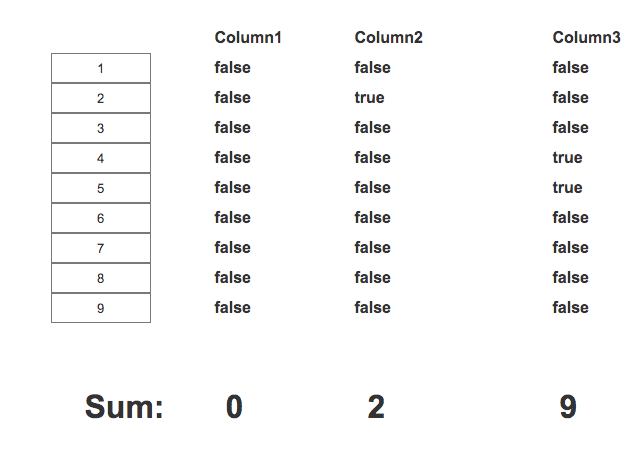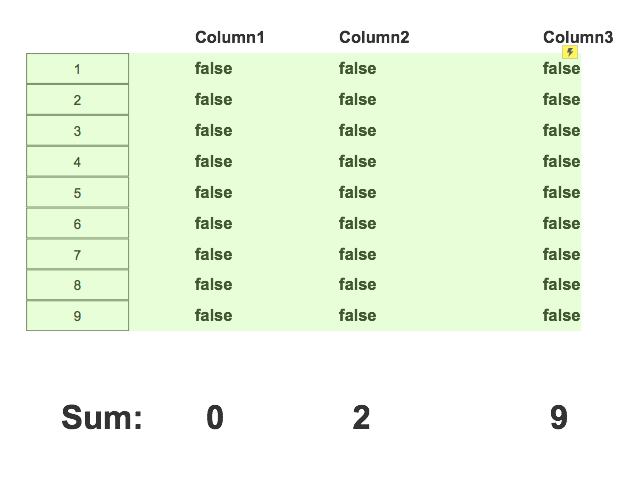# Sum values from repeater based on the value of another cell

#1

Hello,

I`m trying to get the sum of the numbers on the left based on the value of each column (true or false).

I faked the sum on the bottom to demonstrate what I wantSo basically, I need to sum all the values that correspond to the true on the other column.

The 3 columns are in the same repeater:#2

Hi!

You can do the following in OnItemLoad. I’m putting comments inline (which I wish you could do in the product itself). A bit of pseudo code in here as well.

``````OnItemLoad
if (value) [[Item.isFirst]] is equal to true
-- we're on the first row, so zero-out all sums
set text on widgets sum1 and sum1 and sum2 to 0
if true
-- do the normal stuff here, like setting the values of the widgets in the repeater row to the column values
if (value) [[Item.Column1]] is equal to true
set text on widget sum1 to [[Target.text + 1]]
if (value) [[Item.Column2]] is equal to true
set text on widget sum2 to [[Target.text + 1]]
if (value) [[Item.Column3]] is equal to true
set text on widget sum3 to [[Target.text + 1]]
``````

Be careful of IF vs. ELSE IF. (You should have no ELSE IFs here). You can toggle them by right-clicking.)